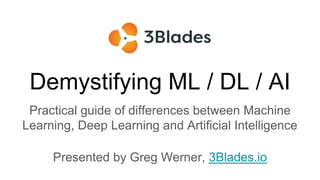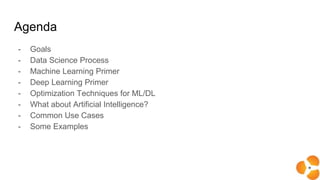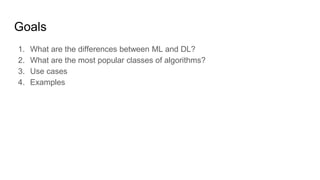Se está descargando tu SlideShare. ×

# Demystifying Ml, DL and AI

Anuncio
Anuncio
Anuncio
Anuncio
Anuncio
Anuncio
Anuncio
Anuncio
Anuncio
Anuncio
AnuncioCargando en…3
×

1 de 21 Anuncio

# Demystifying Ml, DL and AI

This presentation attempts to explain some of the concepts used when describing data science, machine learning, and deep learning. IT also describes data science as a process, rather than as a set of specific tools and services.

This presentation attempts to explain some of the concepts used when describing data science, machine learning, and deep learning. IT also describes data science as a process, rather than as a set of specific tools and services.

Anuncio
Anuncio

### Demystifying Ml, DL and AI

1. 1. Demystifying ML / DL / AI Practical guide of differences between Machine Learning, Deep Learning and Artificial Intelligence Presented by Greg Werner, 3Blades.io
2. 2. Agenda - Goals - Data Science Process - Machine Learning Primer - Deep Learning Primer - Optimization Techniques for ML/DL - What about Artificial Intelligence? - Common Use Cases - Some Examples
3. 3. Goals 1. What are the differences between ML and DL? 2. What are the most popular classes of algorithms? 3. Use cases 4. Examples
4. 4. The Data Science Process Effective ML and DL need a process
5. 5. Data Science Process (cont.) The Processes are actually the same! The difference is in the algorithms and methods used to train and save models.
6. 6. The Data Science Process (cont.)
7. 7. Data Preparation Primer
8. 8. Data Preparation Getting, Cleaning and Preparing Data
9. 9. Data Preparation (cont.) Preprocess Data
10. 10. Data Preparation (cont.) Transform Data
11. 11. Spot Check Algorithms
12. 12. Grouping Algorithms Learning Style
13. 13. Grouping Algorithms By Similarity
15. 15. Deep Learning (cont.) From Jeff Dean (“Deep Learning for Building Intelligent Computer Systems”): “When you hear the term deep learning, just think of a large deep neural net. Deep refers to the number of layers typically and so this kind of the popular term that’s been adopted in the press. I think of them as deep neural networks generally.”
16. 16. Deep Learning (cont.) Automatic feature extraction from raw data, also called feature learning.
18. 18. Deep Learning (cont.) 1. Input a set of training examples 2. For each training example xx, set corresponding input activation and: a. Feedforward b. Output error c. Backpropagate the error 3. Gradient descent
19. 19. Deep Learning (cont.) Deep learning excels with unstructured data sets. Images of pixel data, documents of text data or files of audio data are some examples.
20. 20. Take Aways ● We can’t get around ‘Data Munging’, for now anyway ● ML and DL are actually related. DL is used mostly for supervised and semi-supervised learning problems. ● Automating the ML/DL pipeline and offering collaboration environments to complete all these tasks are necessary.

### Notas del editor

• Data Science Workflow
Define the Problem
What is the problem? Provide formal and informal definitions.
Why does the problem need to be solved? Motivation, benefits, how it will be used.
How would I solve the problem? Describe how the problem would be solved manually to flush domain knowledge.
Prepare Data
Data Selection. Availability, what is missing, what can be removed.
Data Preprocessing. Organize selected data by formatting, cleaning and sampling.
Data Transformation. Feature engineering using scaling, attribute decomposition and attribute aggregation.
Data visualizations such as with histograms.
Spot Check Algorithms
Test harness with default values.
Run family of algorithms across all the transformed and scaled versions of dataset.
View comparisons with box plots.
Improve Results (Tuning)
Algorithm Tuning: discovering the best models in model parameter space. This may include hyper parameter optimizations with additional helper services.
Ensemble Methods: where the predictions made by multiple models are combined.
Feature Engineering: where the attribute decomposition and aggregation seen in data preparation is tested further.
Present Results
Context (Why): how the problem definition arose in the first place.
Problem (Question): describe the problem as a question.
Solution (Answer): describe the answer the the question in the previous step.
Findings: Bulleted lists of discoveries you made along the way that interests the audience. May include discoveries in the data, methods that did or did not work or the model performance benefits you observed.
Limitations: describe where the model does not work.

• Data Selection what data is available, what data is missing and what data can be removed.
Data Preprocessing: organize, clean and sample.
Data Transformation: scaling, attribute decomposition and attribute aggregation.
• This is a subset of the available data that you need to train your ML/DL models.
What is the extent of the data, where is it located and is there anything missing to solve your problem.
Usually, this process is a little more involved with Machine Learning due to the data set types used to train and save Machine Learning models.
With Machine Learning, more is not better, usually.
• Formatting: related to data formats and schemas. ETL tools are great for this step.
Cleaning: cleaning data is the removal or fixing of missing data.
Sampling: sometimes you can get a smaller representation of your data to improve training times.
• Scaling: provide consistency with values between 0 and 1 with standard units of measure.
Decomposition: feature separation. Hour and time is an example.
Aggregation: counts for login instead of full time stamp is an example.
• Test Harness: The goal of the test harness is to be able to quickly and consistently test algorithms against a fair representation of the problem being solved.
Performance Measure: classification, regression or clustering.
Cross Validation: use the entire data set to train your model. In short this is to separate your data into a number of chunks (folds) except one and the final test is done on that fold.
Testing Algorithms: test with groups
• Regression
Regression is actually a loose term because its and algebraic process.
Ordinary Least Squares Regression (OLSR)
Linear Regression
Logistic Regression
Stepwise Regression
Locally Estimated Scatterplot Smoothing (LOESS)

Instance Based
Also called winner-take-all methods and memory-based learning. Focus is put on the representation of the stored instances and similarity measures used between instances.
k-Nearest Neighbor (kNN)
Learning Vector Quantization (LVQ)
Self-Organizing Map (SOM)
Locally Weighted Learning (LWL)
Regularization
Penalizes more complex algorithms.
Ridge Regression
Least Absolute Shrinkage and Selection Operator (LASSO)
Elastic Net
Least-Angle Regression (LARS)
Decision Tree
Often fast and accurate, used for both classification and regression.
Classification and Regression Tree (CART)
Iterative Dichotomiser 3 (ID3)
C4.5 and C5.0 (different versions of a powerful approach)
Chi-squared Automatic Interaction Detection (CHAID)
Decision Stump
M5
Conditional Decision Trees
Bayesian
Used in classification and regression.
Naive Bayes
Gaussian Naive Bayes
Multinomial Naive Bayes
Averaged One-Dependence Estimators (AODE)
Bayesian Belief Network (BBN)
Bayesian Network (BN)
Clustering Algorithms
Organizes data into groups.
k-Means
k-Medians
Expectation Maximisation (EM)
Hierarchical Clustering
Association Rule
Association rule learning methods extract rules that best explain observed relationships between variables in data. Paints relationships between large multi-dimensional data sets.
Apriori algorithm
Eclat algorithm
Artificial Neural Networks (ANN), usually included with Deep Learning
The most popular artificial neural network algorithms are:
Perceptron
Back-Propagation
Hopfield Network

Deep Learning
Used in semi-supervised learning
Deep Boltzmann Machine (DBM)
Deep Belief Networks (DBN)
Convolutional Neural Network (CNN)
Stacked Auto-Encoders

Dimensionality Reduction
Used to visualize dimensional data or to simplify data which can then be used in a supervised learning method.
Principal Component Analysis (PCA)
Principal Component Regression (PCR)
Partial Least Squares Regression (PLSR)
Sammon Mapping
Multidimensional Scaling (MDS)
Projection Pursuit
Linear Discriminant Analysis (LDA)
Mixture Discriminant Analysis (MDA)